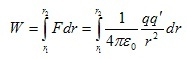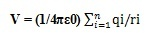# What is Electric Potential Energy : Working & Its Applications

Whenever you move approximately in the gravitational field of the earth, then changing your position within this field is possible by exerting energy. Similarly, electric charges have fields in their region of them. So an electric charge has to do some work if it needs to change its position. Here the work is the electric potential energy or electric energy of the charge. Potential energy is the capacity of doing work that occurs from location or arrangement.

For instance, if a positive charge ‘Q’ is set at some point within space, then any other positive charge near it will face a repulsive force then that will have potential energy. There are different types of potential energies like gravitational, elastic, spring, and electrical. So this article discusses one of the types of potential energies like electric potential energy.

## What is Electric Potential Energy?

Electric potential energy definition is; when an object gains some energy by moving away from the electric field. The electric potential can be obtained for any change by dividing the potential energy by the amount of charge. This is also known as the electrostatic potential or electric field potential.

The electric potential energy of an object mainly depends on two main elements like its own electric charge and relative location through other objects which are electrically charged. This is a scalar quantity that can be measured in terms of Joules & denoted by V, ΔV, ΔU & U. The dimensional formula of electric potential energy is ML^2T^-3A^-1.

There are two common methods of measuring the electric potential energy of any system. The units of common electric potential energy are volts (V) & electron volts (eV). The base units of volts can be simply written as Joules per Coulombs (J/C). An electron volt or eV is the sum of energy an electron gets once the electric potential of a system is enhanced by 1 volt & electron volts (eV) are normally used to measure energy within nuclear & particle physics.

### Electric Potential Energy Formula & Its Derivation

Consider the following system including two-point charges where a positive test charge like q’ moves within the field generated through a fixed point charge like ‘q’ shown in the following figure.

In the above figure, charge ‘q’ at point ‘P’ is fixed and is moved from point ‘R’ to ‘S’ through a PRS radial line that is shown in the above figure.

Let the distance between two points like P & R is ‘r1’ whereas ‘r2’ is the distance between two points like P & S.

The magnitude of the force on a positive test charge can be given through Coulomb’s law is

F = (1/4πε0) qq’/r^2

If charge q’ moves in the direction of ‘S’ throughout a little displacement ‘dr’ then work completed through this force while making the little displacement ‘dr’ is

dW = F.dr

dW = (1/4πε0) qq’/r^2 x dr

The complete work finished throughout this force when test charge moves from R to S point that is from r1 to r2 is,Or W = qq’/4πε0 (1/r1-1/r2)

Therefore the work done for this specific path on q’ test charge mainly depends on finish points, not on the lane taken.

Work completed while moving the q’ test charge from two points R to S is equivalent to the change within potential energy while moving the q’ test charge from two points R to S. So,

W = U(r1) – U(r2)

Where

U(r1) = qq’/4πε0r1 is the electric potential energy of q’ test charge once it is at ‘R’ point.

U(r2) = qq’/4πε0r2 is the electric potential energy of q’ test charge once it is at ‘S’ point.

Therefore, potential energy of q’ test charge at any distance ‘r’ from ‘q’ charge can be given through

U = qq’/4πε0r

The above equation gives the electric potential energy for pair charges which mainly depends on the division between the charges but not on the charged particles’ location.

If we bring the q’ test charge from r2=∞ distance to r1=r distance then we have to do some work from electric forces which is equivalent to enhance in potential energy can be given by the above equation.

Therefore, the test charge’s potential energy at any point within the electric field is the work finished from the electric forces to carry the charge from a large distance to some distance under consideration.

### Electrical Potential Due to a Point Charge

In an electric field, the electric potential at a specific point can be defined as the amount of work completed to move a positive unit charge from infinity to that point through any path once the electrostatic force is applied.

Assume that a +ve charge is located at a point then it will use a force because of the existence of an electric field. So, the electric potential at any end from the positive charge ‘+q’ at ‘r’ distance can be given as

V = (1/4πε0) q/r

Where

‘r’ is the +Ve charge’s position vector.

‘q’ is the source charge.

When the electric potential unit is volt, then 1V = 1 JC^-1

Once work is completed while moving a 1-coulomb charge from infinity to a specific point because of an electric field from the electrostatic force, then it is known as 1V of the electrostatic potential at a specific point.

Similarly, electric potential because of multiple charges can be expressed as;#### Difference between Electric Potential Energy and Electric Potential

Both terms like Electric potential energy and electric potential are related but there are some differences between them which are discussed below.

 Electric Potential Energy Electric Potential Electric potential energy can be defined as the energy required to move a charge from the electric field. An electric potential can be defined as the amount of work completed to move the unit charge from infinity to the fixed point in an electric field. Electric potential energy can be denoted with ‘U’. An electric potential can be denoted with ‘V’. This is also known as electrostatic potential energy. Electric potential is also called voltage. This can be calculated by using this formula like U= kq1q2/r12 where, ‘k’ is the electrostatic charge, q1,q2 are charges and ‘r12’ is the separation between two charges. This can be calculated by using this formula like V= kq/r where ‘k’ is the electrostatic charge, ‘q’ is the charge and ‘r’ is the separation between charges. It is signified with the sum of potential energies because of different charge pairs. It is also signified as an amount of potential at different locations because of the ‘q’ charge. This energy mainly depends on the charge of the object which experiences the electric field. This is completely location-dependent.

The advantages of electric potential energy include the following.

• Nuclear energy is also one type of electric potential energy which is a highly consistent form of energy.
• The electricity generated from nuclear power is fairly low at approximately 3 to 5 c/kwh making it extremely attractive to build hydro plants.
• The cost of this energy is low as compared to other energy sources like gas and coal.

The disadvantage of electric potential energy is, that it depends on the charge of the object in the electric field.

### Applications

The applications of electric potential energy include the following.

• This energy is used to describe potential energy within systems through time-variant electric fields.
• This energy is very helpful in moving a charge against an electric field.
• This energy within electricity is very convenient to use for each unit charge known as voltage or electric potential.

Thus, this is all about an overview of electric potential energy and its derivation with advantages, disadvantages, and applications. Here is a question for you, what is the electric potential difference?